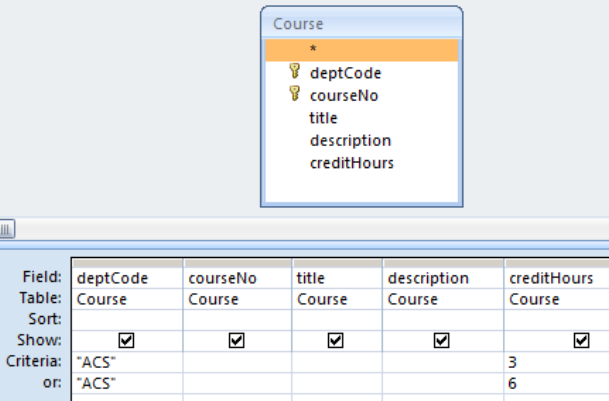# 6.1: Logical Expressions

$$\newcommand{\vecs}{\overset { \rightharpoonup} {\mathbf{#1}} }$$ $$\newcommand{\vecd}{\overset{-\!-\!\rightharpoonup}{\vphantom{a}\smash {#1}}}$$$$\newcommand{\id}{\mathrm{id}}$$ $$\newcommand{\Span}{\mathrm{span}}$$ $$\newcommand{\kernel}{\mathrm{null}\,}$$ $$\newcommand{\range}{\mathrm{range}\,}$$ $$\newcommand{\RealPart}{\mathrm{Re}}$$ $$\newcommand{\ImaginaryPart}{\mathrm{Im}}$$ $$\newcommand{\Argument}{\mathrm{Arg}}$$ $$\newcommand{\norm}{\| #1 \|}$$ $$\newcommand{\inner}{\langle #1, #2 \rangle}$$ $$\newcommand{\Span}{\mathrm{span}}$$ $$\newcommand{\id}{\mathrm{id}}$$ $$\newcommand{\Span}{\mathrm{span}}$$ $$\newcommand{\kernel}{\mathrm{null}\,}$$ $$\newcommand{\range}{\mathrm{range}\,}$$ $$\newcommand{\RealPart}{\mathrm{Re}}$$ $$\newcommand{\ImaginaryPart}{\mathrm{Im}}$$ $$\newcommand{\Argument}{\mathrm{Arg}}$$ $$\newcommand{\norm}{\| #1 \|}$$ $$\newcommand{\inner}{\langle #1, #2 \rangle}$$ $$\newcommand{\Span}{\mathrm{span}}$$$$\newcommand{\AA}{\unicode[.8,0]{x212B}}$$

Sometimes we need to retrieve data based on multiple criteria which are expressed as logical expressions involving the logical operators and, or, and not. For example a student using the University database might want to know which courses offered by the Chemistry and Physics departments are not full courses (that is, they are not 6 credit hour courses). The criteria can be restated with emphasis on logical operators:

• a course is a Chemistry course or a course is a Physics course, and
• the course has any value for credit hours but not 6.

Such criteria involves and, or, and not. Stating the requirements in natural language may seem easy, but stating these properly in the forms-based Query By Example design window requires specialized knowledge.

MS Access provides a way for us to specify the above using the Criteria and Or lines in the Grid. We will consider each of the operators And, Or, and Not.

## AND

If one specifies multiple criteria on one line in the Grid area, these criteria are ANDed. For a row to contribute to the result of the query the row must satisfy all the criteria.

Example

Suppose we want a list of all ACS 3 credit hour courses. We need to obtain the rows in Course where the logical expression

(deptCode="ACS") AND (creditHours=3)

is true. We code this in QBE as:Figure $$\PageIndex{1}$$: Two expressions that are ANDed.

## OR

If one specifies multiple criteria on both the Criteria and Or lines the criteria on each line is ANDed, and those evaluations are then ORed. If for some row either one or both of the sub-expressions evaluate to true, then the row will be selected for display.

Example

Suppose we need a list of all ACS courses that are 3 or 6 credit hour courses. Logically we can express this as:

(deptCode="ACS" AND creditHours=3)

OR

(deptCode="ACS" AND creditHours=6)

We code this in QBE as:Figure $$\PageIndex{2}$$: Two expressions that are ORed.

## NOT

The NOT logical operator negates a logical expression.

Example

To get a list of 3-credit hour courses we would use a criteria of 3, but to list courses that are not 3 credit hours one could use the criteria: NOT 3, which, written in long form is:

NOT (creditHours = 3)

Coding this in QBE we have:Figure $$\PageIndex{3}$$: Using NOT.

## Exercises

Write queries to:

Exercise $$\PageIndex{1}$$

List all courses in Mathematics and Statistics.

Exercise $$\PageIndex{2}$$

List all courses in Mathematics and Statistics where the credit hours are greater than 1.

Exercise $$\PageIndex{3}$$

Lists the titles of courses offered by the Chemistry and Physics departments that are not full courses (that is, they are not 6 credit hour courses).

Exercise $$\PageIndex{4}$$

List all 3 and 6 credit hour courses that are not ACS courses.